# Getting Started with Simulink in MATLAB: Designing a Model

Published  October 29, 2018   0Simulink has becomes most used engineering software package in last few years among academies and industries. Simulink comes integrated with MATLAB software which is used for modelling, simulating and analyzing the dynamic systems. Simulink provides a Graphical User Interface (GUI) as block diagrams to build your model like you are building on using pencil and paper.

To understand Simulink you can consider a simple example,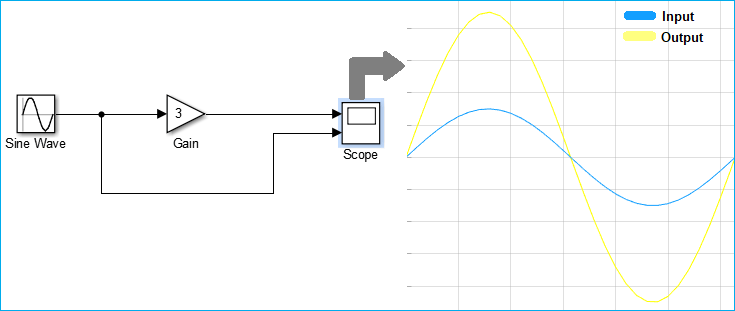Here, in the above example we are generating a sine wave from sine wave block and amplifying it by a gain factor which you directly check on Simulink by double clicking the scope block.

If you are new to MATLAB, first learn the terminologies used in MATLAB and start with LED Blinking.

### How to Launch Simulink in MATLAB?

To open the Simulink in MATLAB, you can just click on the Simulink button from the MATLAB menu bar, as shown in below image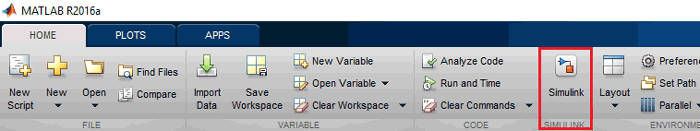Else, you can just use the command window to open Simulink. Just write ‘simulink’ in the command line and hit enter.

`simulink`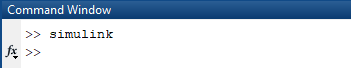Below is the first window which appears after opening Simulink: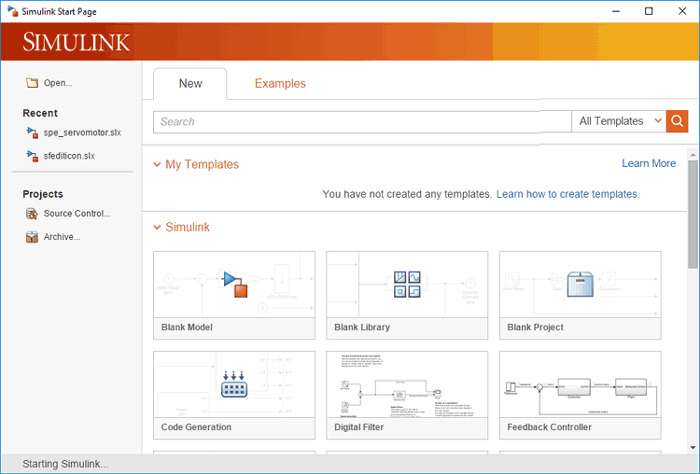As you can see in the image there are different options to do different tasks, like you can create your template, blank model, blank library and many others.

Whenever we need to design a new model, we choose ‘Blank Model’ option by double-clicking on it. The blank model window which we can also called as Simulink window looks like the image below.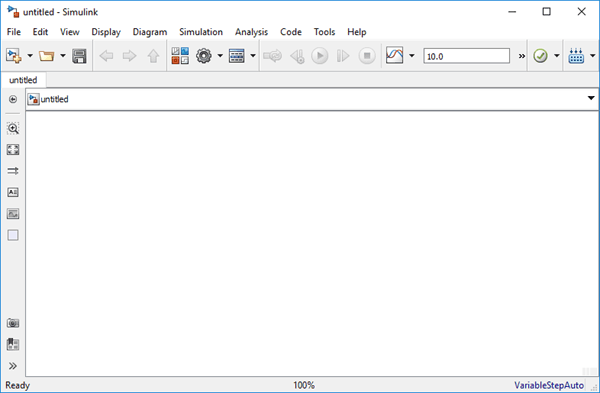Simulink Library Browser contain sinks, sources, connectors, linear and non-linear components. Simulink is far better than the other previous simulation packages that needs to formulate the equations into a program, while in Simulink you can choose the function and blocks and you just have to enter the values of the variable of the equations.

To open a Simulink Library Browser you can simply click on the Library browser button from the Simulink menu, as shown below image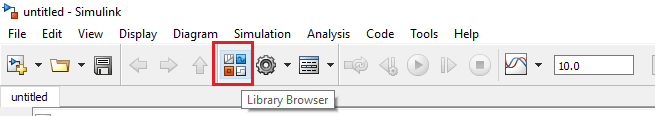The other way to open the Simulink library browser is to type the below command in the command window. The command is case-sensitive so be careful while typing:

`slLibraryBrowser`This is how the Simulink library browser looks, in which you can search for sinks, sources, connectors, linear and non-linear components.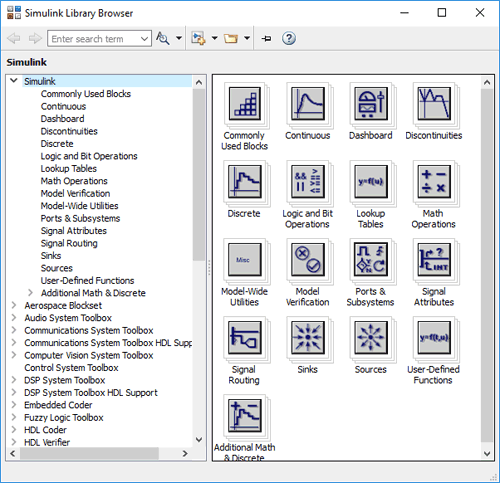### Running Demo Model with MATLAB Simulink

Simulink already contains number of simple and advanced models of different types of systems like audio, communication, computer vision, DSP, real-time and many others, as you can see in the below image,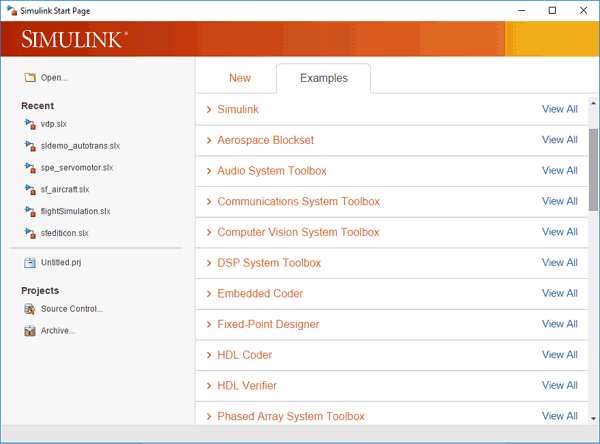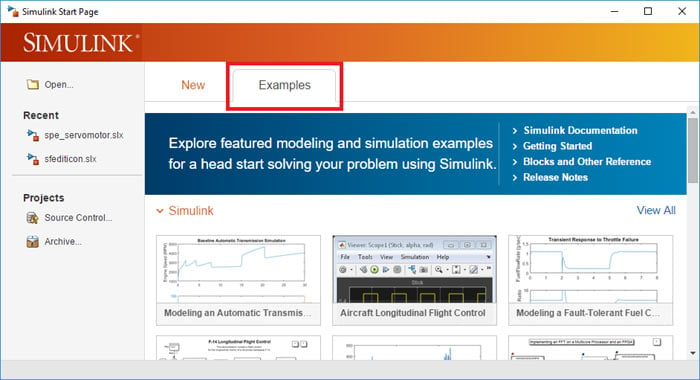To run an example or demo model just double-click on it and a pop-up window of its Simulink model will appear, as shown in below image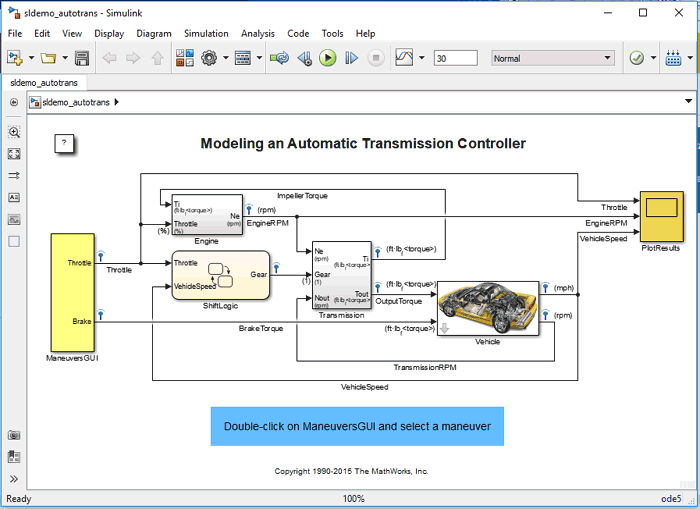After modelling you can simulate the model using a choice of integration method, Simulink menu or command window. The easiest and convenient way is to simply use Simulink ‘RUN’ button from the Simulink menu to run a model in Simulink, as shown in below image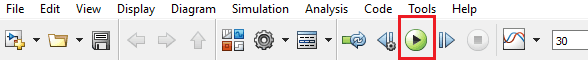You can also able to use or edit the demo model if you want to.

### Starting the Debugger

Simulink Debugger is a tool of Simulink to locate or diagnose bugs in a model. Debugger helps you to check or run the simulation step by step and also displays the input, output and block states.

To start a debugger you just have to click on debug model in the simulation section of the Simulink menu bar, as shown in below image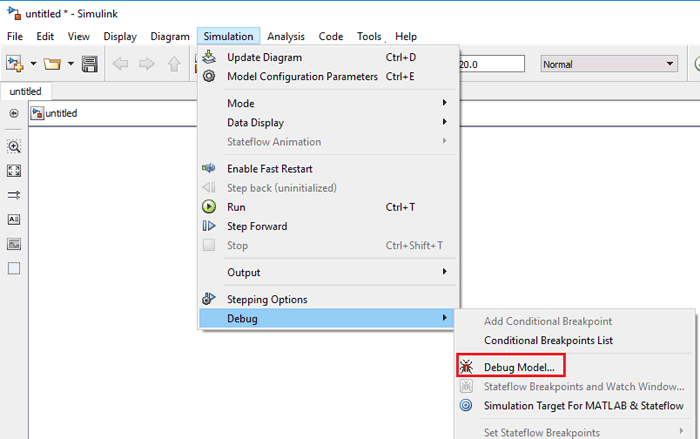You can even start a debugger from the command window just by typing the below command,

`sldebug (‘model name’)`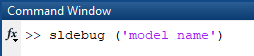### Creating a Simulink Model for Signal Amplifier

Step 2:- Choose the ‘Blank Model’ from the Simulink, as shown in the below imageStep 3:- Now, we are going to build a ‘Signal Amplifier Model’ which takes an input and amplifies it by a gain factor.

For Signal Amplifier Model we need sine wave block, gain block and scope.

Step 4:- Open the library browser by typing ‘sl’LibraryBrowser’ in the command window. And, choose the required blocks and drag them into the Simulink window from library browser window, as shown in below image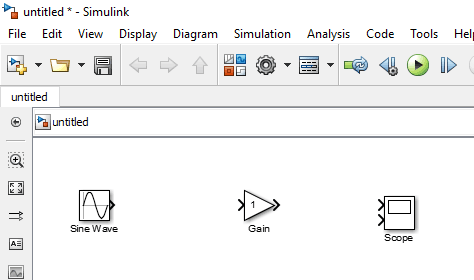Step 5:- After placing all the blocks into the Simulink window, you have to connect them according to the image shown below,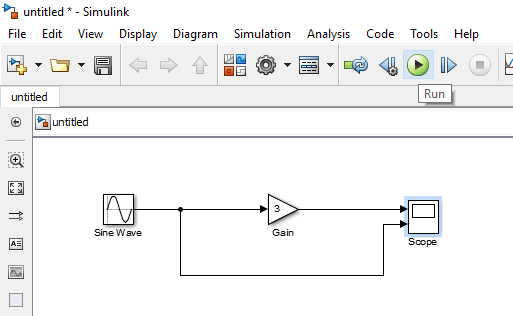Step 6:- You have to change the setting for getting two inputs in scope, so double click on scope and then go to setting and change ‘Number of input Ports’ to ‘2’, as shown in below image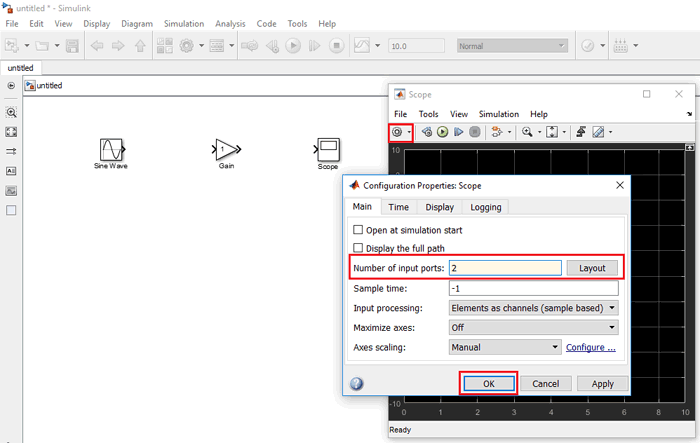Step 7:- Now, for amplification, you can set the value of amplitude of sine wave and gain factor by double clicking on the respective block.

Step 8:- After completing this, we can run our Simulink model from the ‘RUN’ button given in the Simulink menu.Wait till Simulink compile your model, which you can see in the bottom right corner of the window.

Step 9:- Now, for result double-click on scope and you will be able to see both input and amplified waveform, as shown in image below,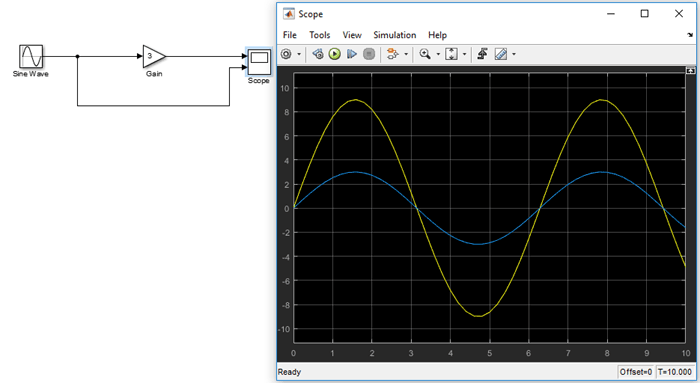As a result, if you observe the output waveform, it is amplified by a factor of 3.

Step 10:- Now, you can save your model and can also get a print out, the extension for Simulink design is ‘.slx’ so keep an eye on extension while saving, generally it automatically takes the ‘.slx’ extension.

You can also check the video given at the end for ‘Creating a Model using MATLAB Simulink’.

### Modelling a Dynamic Control System

Simulink is used to analyze various real-time-based complex systems related to friction, air resistance, gear slippage etc. These system are very complex, and to design a Model for that is out of the scope the article. But to learn how to model dynamic control system, MATLAB itself provided a nice documentation. You can access that in Getting Started tutorial of the MATLAB. Go to ‘Model a Dynamic System’ after selecting Getting Started.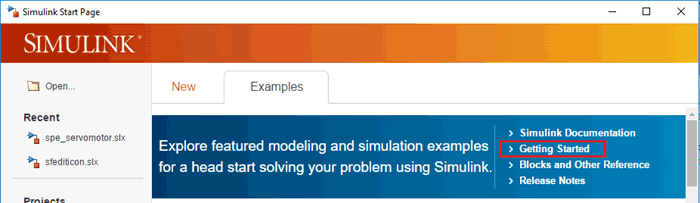You will be redirected to the help browser of the MATLAB, as shown in below image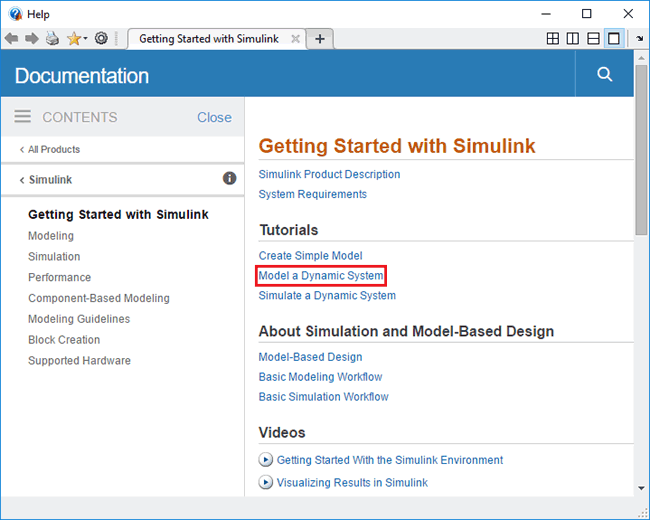The complete process for desiging a Simulink model is shown in the Video below: